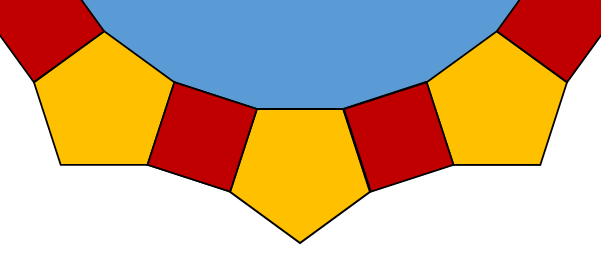# Exam-Style Questions.

## Problems adapted from questions set for previous Mathematics exams.

### 1.

GCSE Higher

The exterior angle of a regular polygon is 72°

What is the name of the regular polygon?

### 2.

GCSE Higher

The diagram, not drawn to scale, show a polygon with one line of symmetry, AE.

Angle HAB is 90° and interior angle DEF is 220°.

Work out the size of angle ABC which is half the size of interior angle BCD and twice the size of angle CDE.### 3.

GCSE Higher

A number of regular pentagons and squares are arranged around the outside of a large blue regular polygon. Just the lower part of the arrangement is shown below.

How many sides does the large blue regular polygon have?### 4.

GCSE Higher

PQRS is a rhombus and C is the midpoint of QS.

The angle QRS is 120o and A is the point on QS such that PA = AQ.

Calculate the size of angle CPA.### 5.

IGCSE Extended

The diagrams are not drawn to scaleABCDEF is a hexagon with AB parallel to ED and BC parallel to FE. GFE and GABH are straight lines. Angle CBH = 70o and angle EFA = 90o. Calculate the values of: (a) $$p$$; (b) $$q$$; (c) $$t$$; (d) $$x$$. K, P, J and N are points on a circle and KN = NP. KJ is a diameter and LKM is the tangent to the circle at K. Angle LKN = 59o. Find the values of: (e) $$y$$; (f) $$z$$.

If you would like space on the right of the question to write out the solution try this Thinning Feature. It will collapse the text into the left half of your screen but large diagrams will remain unchanged.

The exam-style questions appearing on this site are based on those set in previous examinations (or sample assessment papers for future examinations) by the major examination boards. The wording, diagrams and figures used in these questions have been changed from the originals so that students can have fresh, relevant problem solving practice even if they have previously worked through the related exam paper.

The solutions to the questions on this website are only available to those who have a Transum Subscription.

Exam-Style Questions Main Page

Search for exam-style questions containing a particular word or phrase:

To search the entire Transum website use the search box in the grey area below.